##### Actions

Adaptive sampling [a1] is a probabilistic algorithm invented by M. Wegman (unpublished) around 1980. It provides an unbiased estimator of the number of distinct elements (the "cardinality" ) of a file (a sequence of data items) of potentially large size that contains unpredictable replications. The algorithm is useful in data-base query optimization and in information retrieval. By standard hashing techniques [a3], [a6] the problem reduces to the following.

A sequence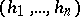of real numbers is given. The sequence has been formed by drawing independently and randomly an unknown numberof real numbers from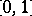, after which the elements are replicated and permuted in some unknown fashion. The problem is to estimate the cardinality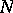in a computationally efficient manner.

Three algorithms can perform this task.

1) Straight scan computes incrementally the sets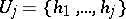, where replications are eliminated on the fly. (This can be achieved by keeping the successivein sorted order.) The cardinality is then determined exactly by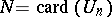but the auxiliary memory needed is, which may be as large as, resulting in a complexity that is prohibitive in many applications.

2) Static sampling is based on a fixed sampling ratio, where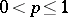(e.g.,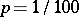). One computes sequentially the samples. The cardinality estimate returned is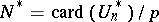. The estimator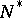is unbiased and the memory used is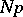on average.

3) Adaptive sampling is based on a design parameter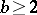(e.g.,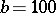) and it maintains a dynamically changing sampling rateand a sequence of samples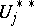. Initially,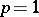and. The rule is like that of static sampling, but withdivided byeach time the cardinality ofwould exceed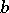and withmodified accordingly in order to contain only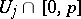. The estimator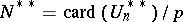(where the final value ofis used) is proved to be unbiased and the memory used is at most.

The accuracy of any such unbiased estimator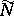ofis measured by the standard deviation ofdivided by. For adaptive sampling, the accuracy is almost constant as a function ofand asymptotically close toa result established in [a1] by generating functions and Mellin transform techniques. An alternative algorithm, called probabilistic counting [a2], provides an estimator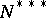of cardinalities that is unbiased only asymptotically but has a better accuracy, of about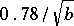.

Typically, the adaptive sampling algorithm can be applied to gather statistics on word usage in a large text. Well-designed hashing transformations are then known to fulfill practically the uniformity assumption [a4]. A general perspective on probabilistic algorithms may be found in [a5].